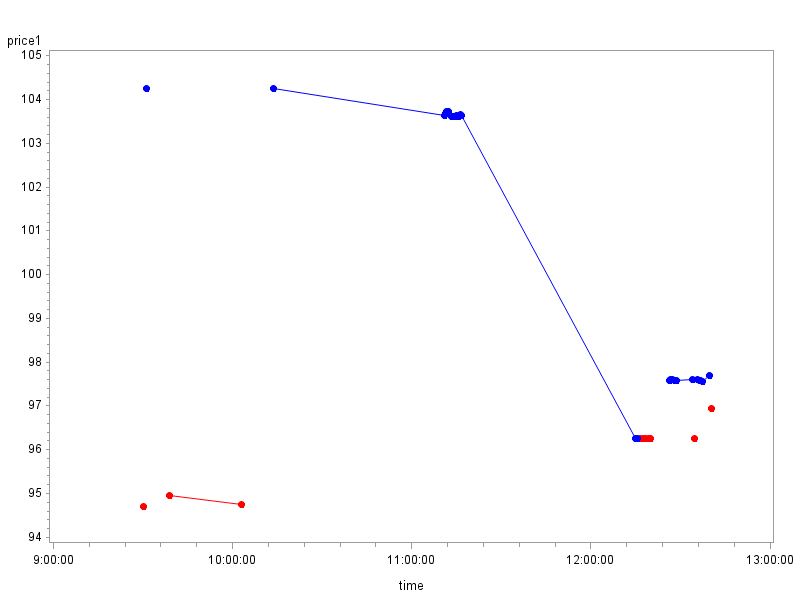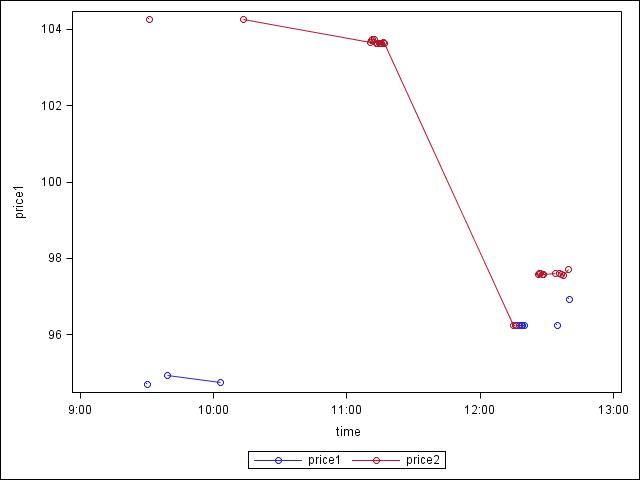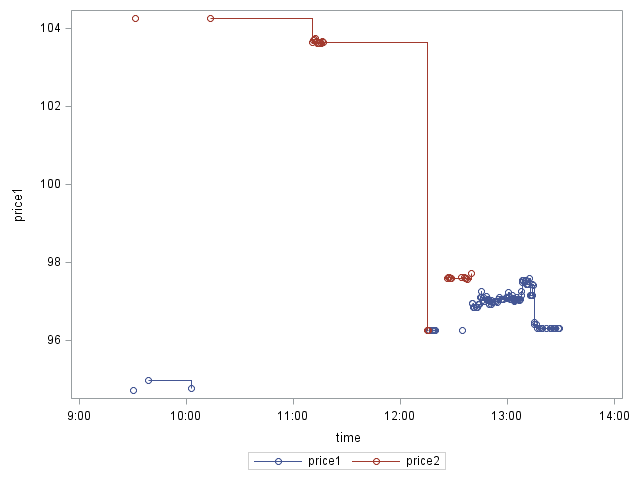## plotting two time series that shows alternatively using proc gplot

I want to plot two time series and interpolate those points that show together. The two time series are part of one original series. The difficulty here is that the two time series shows up alternatively. For example, the first series may be from 8:30 to 9:30 then the second series show up from 9:30 to 10:30 and so on  so forth. I only want to interpolate the points that belong to the same series and show up consecutively.  Simply using symbol statement is problematic because it will interpolate all data points that belong to the same series altogether. I hope I make myself clear. Thank you for your help.

1 ACCEPTED SOLUTION

Accepted Solutions

## Re: plotting two time series that shows alternatively using proc gplot

Here's how to do a graph similar to PG's, but using Proc Gplot ...

symbol1 value=dot interpol=join color=red;

symbol2 value=dot interpol=join color=blue;

proc gplot data=ex;

plot price1*time=1 price2*time=2 / overlay skipmiss;

run;15 REPLIES 15PGStats
Opal | Level 21

## Re: plotting two time series that shows alternatively using proc gplot

If you post some data, I or somebody else will show you how to do this with SGPLOT.

PG

PG

## Re: plotting two time series that shows alternatively using proc gplot

Thank you PG. Here is a example of my data.PGStats
Opal | Level 21

## Re: plotting two time series that shows alternatively using proc gplot

Your time series already have missing values. Is this what you need?

data ex;

infile "&sasforum\datasets\example.txt" firstobs=2;

input Obs time :time10. price1 price2;

format time time10.;

run;

proc sgplot data=ex;

series x=time y=price1 / markers break lineattrs=(pattern=solid) markerattrs=(symbol=circle);

series x=time y=price2 / markers break lineattrs=(pattern=solid) markerattrs=(symbol=circle);

xaxis type=time;

run;PG

PG

## Re: plotting two time series that shows alternatively using proc gplot

Thank you PG. That is what i need. But can i do it with proc gplot?

## Re: plotting two time series that shows alternatively using proc gplot

PG, the missing value is because the two series used to belong one original series. The two are the two parts of one complete series.

## Re: plotting two time series that shows alternatively using proc gplot

Here's how to do a graph similar to PG's, but using Proc Gplot ...

symbol1 value=dot interpol=join color=red;

symbol2 value=dot interpol=join color=blue;

proc gplot data=ex;

plot price1*time=1 price2*time=2 / overlay skipmiss;

run;## Re: plotting two time series that shows alternatively using proc gplot

Thank you Robert. I tried your code. It works. But I want to use interpol=steplj  to link those points. Then it does not work. As you can see these are stock price series. The market is a limit order market where price lasts as long as it is not replaced with better ones.## Re: plotting two time series that shows alternatively using proc gplot

Ahh! - You didn't mention that before!Per the Gplot documentation for the 'skipmiss' option:

SAS/GRAPH(R) 9.4: Reference, Third Edition

Unfortunately ... "The SKIPMISS option is available only with JOIN interpolation."

## Re: plotting two time series that shows alternatively using proc gplot

Sorry Robert, is there any possibility I can do this with interpol=steplj?

## Re: plotting two time series that shows alternatively using proc gplot

Per PG's method, it is possible to do this with proc sgplot.

proc sgplot data=example;

step x=time y=price1/markers break;

step x=time y=price2/markers break;

title;

footnote;

run;## Re: plotting two time series that shows alternatively using proc gplot

You can use gplot's "skipmiss" option to accomplish this.

Just make sure there is at least 1 'missing value' between each section of the line where you want the break to be.

## Re: plotting two time series that shows alternatively using proc gplot

Thank you Robert. Unfortunately, I have a huge amount of data. Inserting a missing data every time there is a alternation is too burdernsome. Is there any other way I can do this using gplot?

## Re: plotting two time series that shows alternatively using proc gplot

also, Robert, I need to plot time series of two prices that show alternatively. The missing value you mentioned need to be the missing values of the price series. I don't know how i can insert missing value for these two series.

## Re: plotting two time series that shows alternatively using proc gplot

When should there be a break in the line? (ie, what is the logic/criteria for making that decision?)

Discussion stats
• 15 replies
• 1788 views
• 8 likes
• 3 in conversation